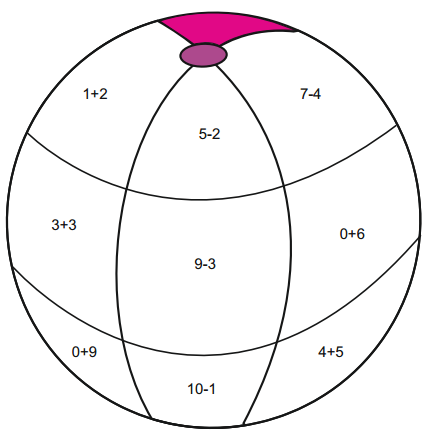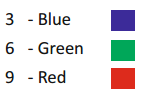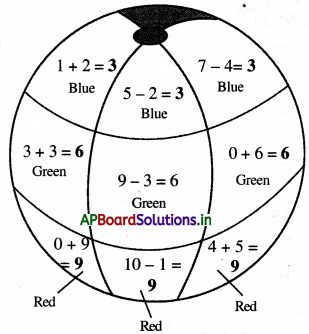# AP Board 1st Class Maths Solutions 9th Lesson How many?

Andhra Pradesh AP Board 1st Class Maths Solutions 9th Lesson How many? Textbook Exercise Questions and Answers.

## AP State Syllabus 1st Class Maths Solutions Chapter 9 How many?

Textbook Page No. 87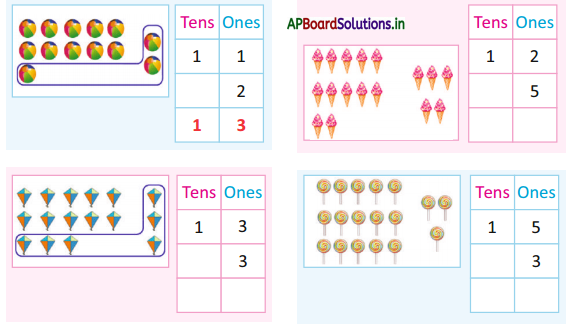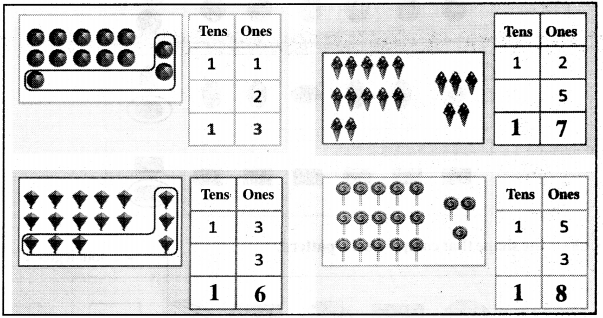Textbook Page No. 88

Add the number given using the bundles of sticks and the loose sticks.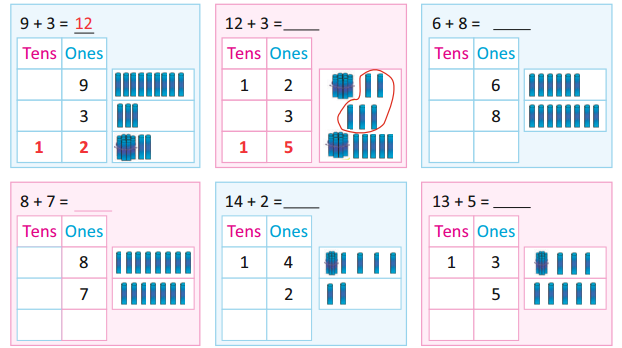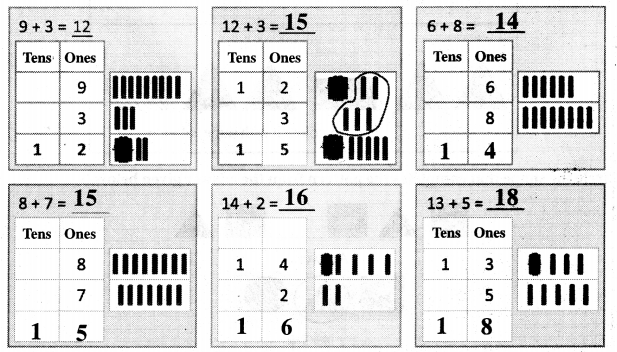Add the numbers using the number line.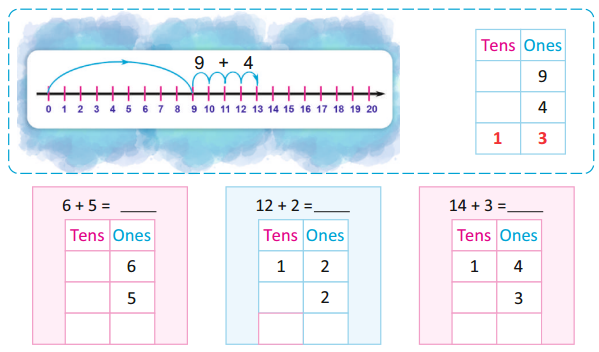Textbook Page No. 89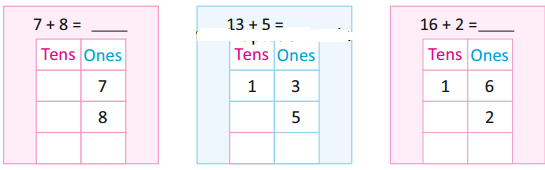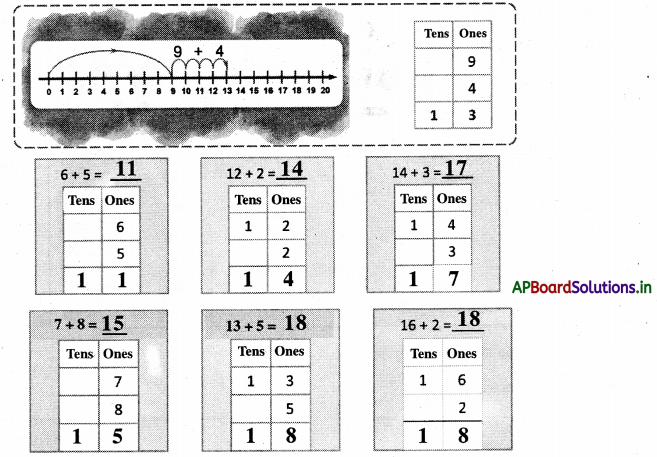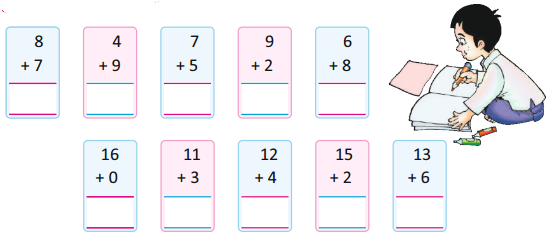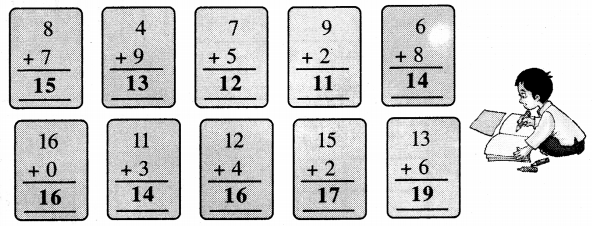Add the numbers given and write in the blank.

Ex. 8 + 6 =          14
6 + 7 =          13
9 + 6 =          15
7 + 8 =          15
5 + 6 =          11
9 + 5 =          14
11 + 4 =          14
0 + 13 =          13
15 + 3 =          18
12 + 0 =          12
3 + 14 =          17
16 + 2 =          18

Textbook Page No. 91, 92

Subtraction :She gave ₹ 10 to the shopkeeper. How much does she get back ?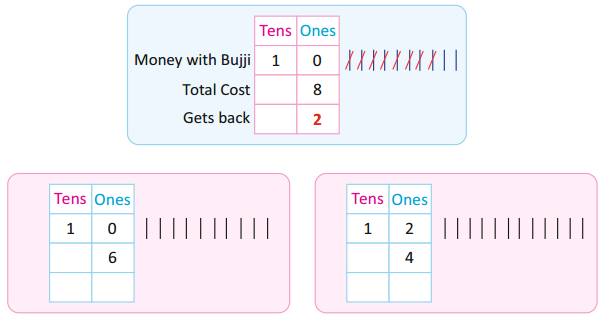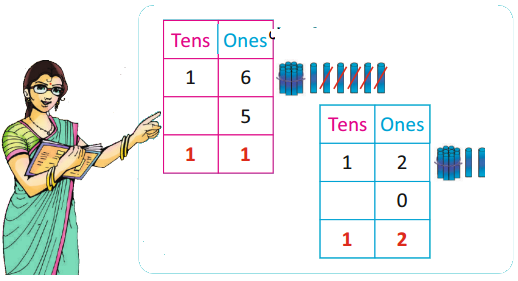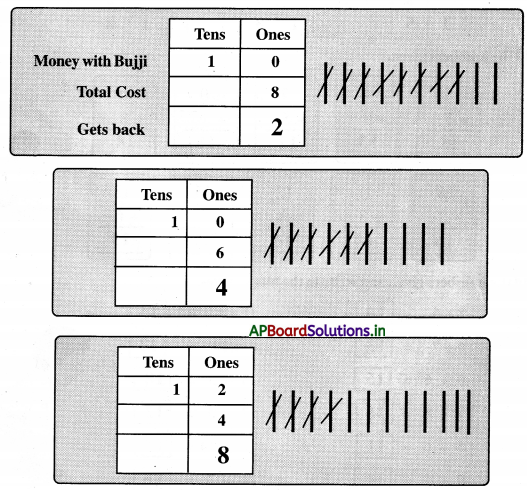Subtracting by drawing lines and crossing out. One is done for you.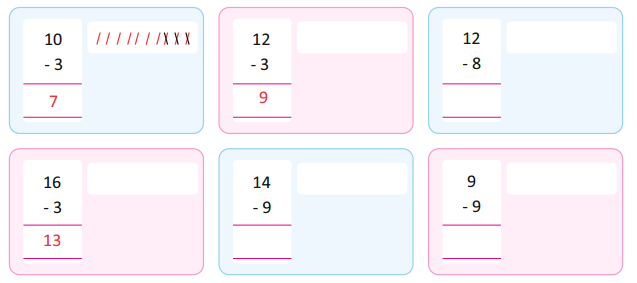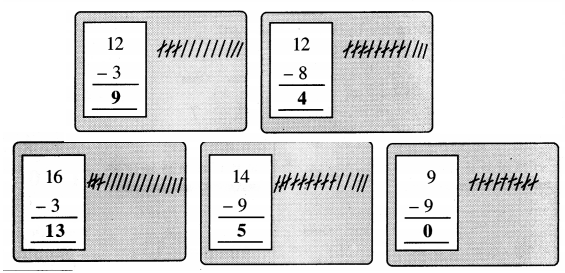Try this: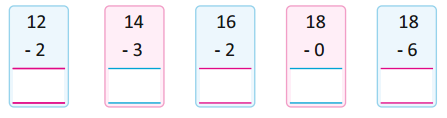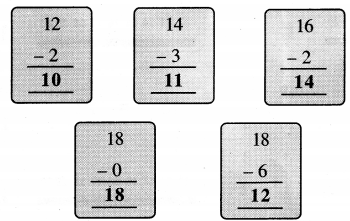Textbook Page No. 93

Subtraction by crossing out.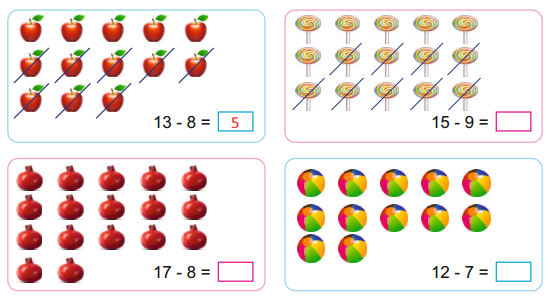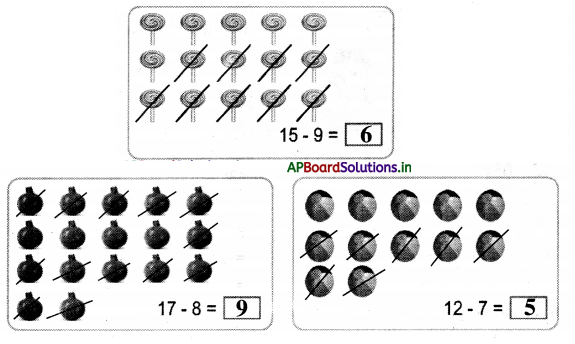Subtraction by counting backward.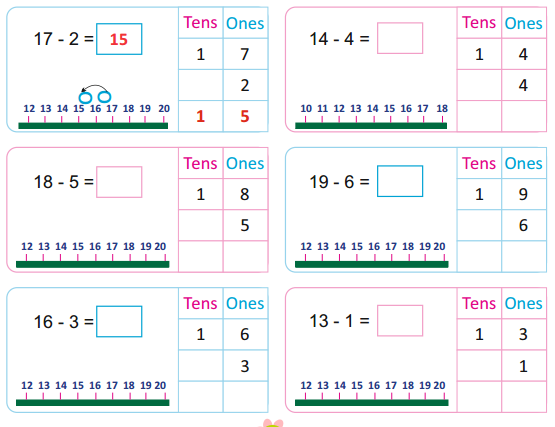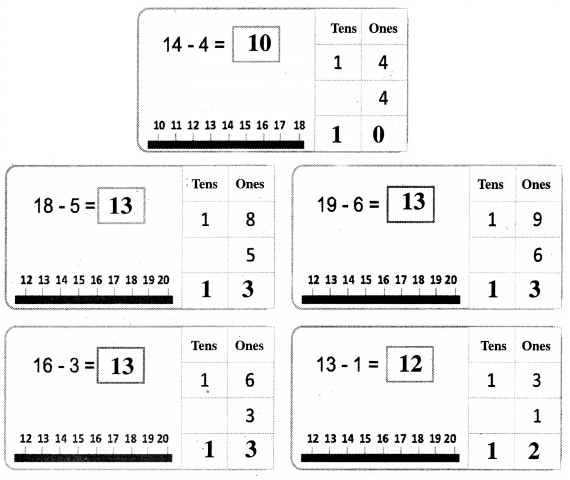Textbook Page No. 94

Word Problems:

Question 1.
Janu had 17 pencils. She gave away 10 pencils to her sister. How many pencils are left with Janu ?
No. of Pencils with Janu = 17
No. of Pencils given to Janu’s sister = 10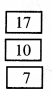No. of Pencils with Janu now = 7Question 2.
There are 15 children in the garden. 8 are girls and the rest are boys. How many are boys ?
No. of Children = 15
No. of Girls = 8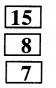No. of Boys = 7

Question 3.
Vidya bought 13 eggs. 7 eggs were broken. How many eggs are left with Vidya ?
No. of eggs bought = 13
No. of eggs broken = 7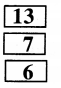No. of eggs with Vidya now = 6Write the number that comes before / after / between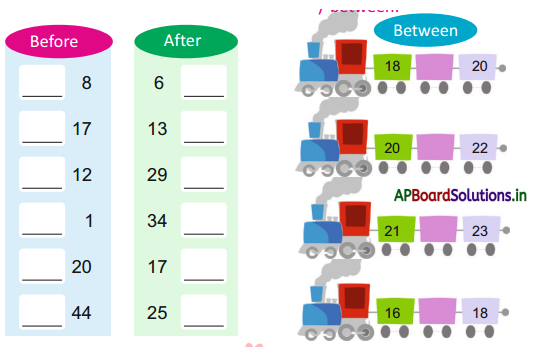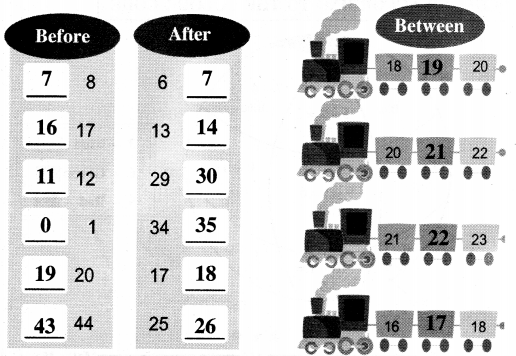Textbook Page No. 95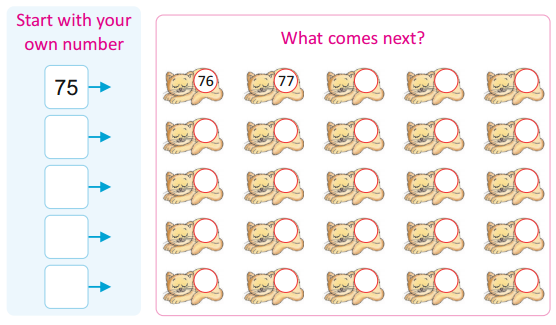Student can take / start with any number of his/her wish & write the following four numbers.Textbook Page No. 96

Colour According To The Colour Code